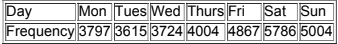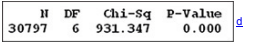×
Get Full Access to Elementary Statistics - 12 Edition - Chapter 11.3 - Problem 1 re
Get Full Access to Elementary Statistics - 12 Edition - Chapter 11.3 - Problem 1 re

×

# Solved: Auto Fatalities The table below lists autoISBN: 9780321836960 18

## Solution for problem 1 RE Chapter 11.3

Elementary Statistics | 12th Edition

• Textbook Solutions
• 2901 Step-by-step solutions solved by professors and subject experts
• Get 24/7 help from StudySoup virtual teaching assistantsElementary Statistics | 12th Edition

4 5 1 394 Reviews
10
0
Problem 1 RE

Auto Fatalities The table below lists auto fatalities by day of the week for a recent year (based on data from the Federal Highway Administration).

Minitab results are also shown. Use a 0.01 significance level to test the claim that auto fatalities occur on the different days of the week with the same frequency. Can you provide an explanation for the results?MINITABStep-by-Step Solution:

Step 1 of 2

The expected values for each category, Ei , could be determined. With these observed and expected numbers of cases, the hypotheses can be written as

H0 : the claim that auto fatalities occur on the different days of the week with the same frequency

H1 : the claim that auto fatalities occur on the different days of the week with the different frequency.

Use a 0.05 significance level

 SL. No. O p = 1/7 E = np (O - E )2 (O - E)2/E 1 3797 0.142857 4399.571 363092.3 82.52902 2 3615 0.142857 4399.571 615552.3 139.9119 3 3724 0.142857 4399.571 456396.8 103.7366 4 4004 0.142857 4399.571 156476.8 35.56636 5 4867 0.142857 4399.571 218489.5 49.66153 6 5786 0.142857 4399.571 1922184 436.9026 7 5004 0.142857 4399.571 365333.9 83.03852 Sum 30797 1 30797 4097526 931.3466

The first step in conducting the significance test is to compute the expected frequency for each outcome given that the null hypothesis is true. For example, the expected frequency of a "1" is 7 since the probability of a "1" coming up is 1/7 and there were a total of 30797.

Expected frequency (E) = np

= 30797(1/7)

= 4399.571

The calculation continues as follows. Letting E be the expected frequency of an outcome and O be the observed frequency of that outcome.

Step 2 of 2

## Discover and learn what students are asking

Calculus: Early Transcendental Functions : The Natural Logarithmic Function: Integration
?In Exercises 1-26, find the indefinite integral. $$\int \frac{10}{x} d x$$

Calculus: Early Transcendental Functions : Differentiation and Integration of Vector-Valued Functions
?Differentiation of Vector-Valued Functions In Exercises 1-6, find r’(t), $$\mathrm{r}\left(t_{0}\right)$$, and $$\mathrm{r’}\left(t_{0}\right)$$ for t

Calculus: Early Transcendental Functions : Introduction to Functions of Several Variables
?In Exercises 7-18, find and simplify the function values. $$f(x, y)=2 x+y^{2}$$ (a) $$\frac{f(x+\Delta x, y)-f(x, y)}{\Delta x}$$

Statistics: Informed Decisions Using Data : Two-Way Analysis of Variance
?To graphically display the role interaction plays in a factorial design, we draw ______.

Statistics: Informed Decisions Using Data : Inference about the Difference between Two Medians: Dependent Samples
?In Problems 3–10, use the Wilcoxon matched-pairs signedranks test to test the given hypotheses at the a = 0.05 level of significance. The dependent sa

Unlock Textbook Solution

Enter your email below to unlock your verified solution to:

Solved: Auto Fatalities The table below lists auto